# Quantum Computing 2 -- Quantum gates (single qubit)

Quantum gates are the elementary operations for the qubits of a quantum computer. They are comparable to the logic gates for classical bits. In contrast to the Boolean operators, all quantum gates are reversible, so there is always an inverse operation that can undo all computation steps. There is also a much wider variety of possible operations for qubits.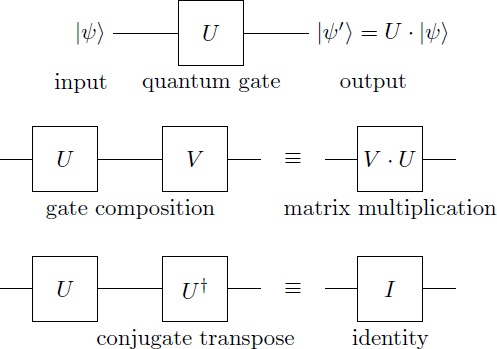A quantum gate for a single qubit is described by a unitary matrix: $U = \left( \begin{array}{cc} a & b \\ c & d \end{array} \right)\, \quad a,b,c,d \in \mathbb{C}$ The operation on a state $| \psi \rangle = \alpha | 0 \rangle + \beta | 1 \rangle$ corresponds to the multiplication of the matrix $U$ with the state vector: \begin{aligned} U |\psi \rangle &= U (\alpha |0\rangle + \beta |1\rangle)\\ &= \left( \begin{array}{cc} a & b \\ c & d \end{array} \right) \left( \begin{array}{c} \alpha \\ \beta \end{array} \right) \\ &= \left( \begin{array}{c} a \alpha + b \beta \\ c \alpha + d \beta \end{array} \right) \\ &= (a \alpha + b \beta) |0\rangle + (c \alpha + d \beta) |1\rangle \end{aligned} Executing two operators $U$ and \ (V ) one after the other is equivalent to an operator resulting from the matrix multiplication of $U$ and $V$: \begin{aligned} V \cdot U &= \left( \begin{array}{cc} a' & b' \\ c' & d' \end{array} \right) \cdot \left( \begin{array}{cc} a & b \\ c & d \end{array} \right) \\ &= \left( \begin{array}{cc} a' a + b' c & a' b + b' d \\ c' a + d' c & c' b + d' d \end{array} \right) \end{aligned} Since the operators are unitary matrices $U$, the adjoint matrix $U ^ \dagger$ corresponds to the inverse operator. (The quantum gate $U ^ \dagger$ thus reverses the effect of the gate $U$.) \begin{aligned} U^\dagger &= \left( \begin{array}{cc} a^\ast & c^\ast \\ b^\ast & d^\ast \end{array} \right) \\ U^\dagger \cdot U &= I = \left( \begin{array}{cc} 1 & 0 \\ 0 & 1 \end{array} \right) \end{aligned} where $I$ is the identity operator or unit matrix and $z^\ast$ denotes the complex conjungate of $z$.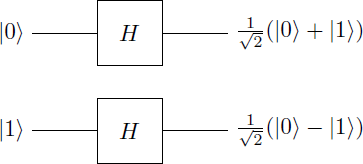The Hadamard gate is one of the most fundamental operations in quantum computing. The Hadamard gate for single qubit is described in the $\{| 0 \rangle, | 1 \rangle \}$ basis by the following matrix:

\begin{aligned} H &= \frac{1}{\sqrt{2}} \left( \begin{array}{cc} 1 & 1 \\ 1 & - 1 \end{array} \right) \\ H|0\rangle &= \frac{1}{\sqrt{2}}(|0 \rangle + |1 \rangle) \equiv |+\rangle\\ H|1\rangle &= \frac{1}{\sqrt{2}}(|0 \rangle - |1 \rangle) \equiv |-\rangle \end{aligned} The basis states $| 0 \rangle$ and $| 1 \rangle$ are converted by the Hadamard gate into a superposition. You can also understand this operation as a transition between the bases $\{| 0 \rangle, | 1 \rangle \}$ and $\{| + \rangle, | - \rangle \}$. The Hadamard matrix is self-adjoint ($H ^ \dagger = H$), so that the two-time application of the Hadamard gate gives the identity ($H \cdot H = H ^ \dagger \cdot H = I$ ).

Phase-Shift

The phase-shift $R_ \phi$ is a whole family of single-qubit gates. It is described by the following matrix in the $\{| 0 \rangle, | 1 \rangle \}$ basis: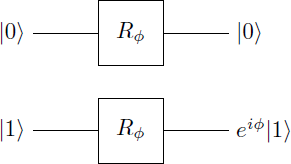\begin{aligned} R_\phi &= \left( \begin{array}{cc} 1 & 0 \\ 0 & \exp(i \phi) \end{array} \right) \\ R_\phi|0\rangle &= |0\rangle \\ R_\phi|1\rangle &= \exp(i \phi) |1\rangle \end{aligned} where $\exp (i \phi) = \cos (\phi) + i \sin (\phi)$. This operation leaves the amplitudes of the state vector unchanged and only introduces a phase shift between the vector components by the angle $\phi \in [- \pi, \pi]$. The adjoint matrix yields $R_ \phi ^ \dagger = R _ {- \phi}$ such that $R _ {- \phi} \cdot R_ \phi = I$.

Of particular importance are the phase shift gates $T = R _ {\pi / 4}$, $S = R _ {\pi / 2}$ and $Z = R_ \pi$.

Pauli-Gates

The Pauli gates are a family of three operators with the matrices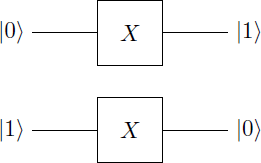\begin{aligned} X &= \left(\begin{array}{cc}0 & 1 \\ 1 & 0 \end{array}\right) \\ Y &= \left(\begin{array}{cc}0 & -i \\ i & 0 \end{array}\right) \\ Z &= \left(\begin{array}{cc}1 & 0 \\ 0 & -1 \end{array}\right) \end{aligned} The $X$ gate is the quantum mechanical equivalent to the classical NOT operator and interchanges the basis states so that $X | 0 \rangle = | 1 \rangle$ and $X | 1 \rangle = | 0 \rangle$ (bit-flip). The $Z$ gate performs a special phase-shift operation around the angle $\pi$ (phase-flip). The $Y$ gate is a combination of bit and phase flip. The Pauli matrices are self-adjoint and obey the algebra $X \cdot X = Y \cdot Y = Z \cdot Z = -i X \cdot Y \cdot Z = I$.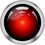Note by Markus Michelmann
3 years ago

This discussion board is a place to discuss our Daily Challenges and the math and science related to those challenges. Explanations are more than just a solution — they should explain the steps and thinking strategies that you used to obtain the solution. Comments should further the discussion of math and science.

When posting on Brilliant:

• Use the emojis to react to an explanation, whether you're congratulating a job well done , or just really confused .
• Ask specific questions about the challenge or the steps in somebody's explanation. Well-posed questions can add a lot to the discussion, but posting "I don't understand!" doesn't help anyone.
• Try to contribute something new to the discussion, whether it is an extension, generalization or other idea related to the challenge.

MarkdownAppears as
*italics* or _italics_ italics
**bold** or __bold__ bold
- bulleted- list
• bulleted
• list
1. numbered2. list
1. numbered
2. list
Note: you must add a full line of space before and after lists for them to show up correctly
paragraph 1paragraph 2

paragraph 1

paragraph 2

[example link](https://brilliant.org)example link
> This is a quote
This is a quote
    # I indented these lines
# 4 spaces, and now they show
# up as a code block.

print "hello world"
# I indented these lines
# 4 spaces, and now they show
# up as a code block.

print "hello world"
MathAppears as
Remember to wrap math in $$ ... $$ or $ ... $ to ensure proper formatting.
2 \times 3 $2 \times 3$
2^{34} $2^{34}$
a_{i-1} $a_{i-1}$
\frac{2}{3} $\frac{2}{3}$
\sqrt{2} $\sqrt{2}$
\sum_{i=1}^3 $\sum_{i=1}^3$
\sin \theta $\sin \theta$
\boxed{123} $\boxed{123}$

Sort by:

Thanks for posting these, as well as the problems. It's cool to realize that this stuff is actually somewhat accessible to non-specialists.

- 3 years ago

Can anyone please tell me the latex code to write example in notes as in wiki.

- 2 years, 9 months ago